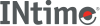﻿ powINtime SDK Help
pow

Computes a value raised to the power of another value.

```#include <math.h>

double pow (double x, double y);
```

#### Parameters

`x`
Number to be raised.
`y`
Power to raise `x` to.

#### Remarks

pow does not recognize integral double values greater than 2 to the 64th power, such as 1.0E100.

#### Return Values

The value of `'x'` raised to the power of `'y'`.

1 if `x` is not 0.0 and `y` is 0.0.

0, and the function sets errno to EDOM if `x` is 0.0 and `y` is negative.

0, and the function sets errno to EDOM and prints a DOMAIN error message to stderr if both `x` and `y` are 0.0, or if `x` is negative and `y` is not an integer.

+/-HUGE_VAL, and sets errno to ERANGE if an overflow results. No message is printed on overflow or underflow.

This function does not return standard ANSI domain or range errors.

#### Requirements

Versions Defined in Include Link to
INtime 3.0 intime/rt/include/math.h math.h clib.lib